# Siegel disc

Consider a holomorphic vector field with a singularity, i.e. of the formwith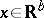or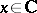. The eigenvalues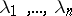are said to be resonant if among the eigenvalues there exists a relation of the form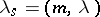, where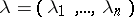,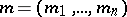with,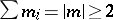and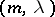is the usual inner product. H. Poincaré proved in his dissertation that if the eigenvalues ofare non-resonant, then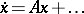can be reduced to the linear equation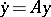by a formal change of variable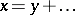. The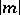th order term of this change of variable is given in terms of lower-order terms divided by a term of the form. Since, in general, such terms can be either zero (in the resonant case) or arbitrarily close to zero (in which case one says that one has small denominators), the power seriesin general does not converge. An example that divergence can occur was already given by L. Euler. The eigenvalue vectorbelongs to the Poincaré domain if zero is not in the convex hull of the eigenvalues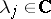; its complement is called the Siegel domain. When the vector of eigenvaluesis in the Poincaré domain, the vector field can be reduced to a polynomial vector field (called the normal form) by a biholomorphic change of variables in a neighbourhood of the singularity. If, in addition, there are no resonances, then one can choose the polynomial normal form to be linear. Ifis in the Siegel domain, then one says it satisfies a Diophantine condition if there exist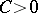,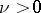such that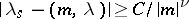for all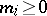with. Siegel's theorem states that, in this case, the vector field can be reduced to its linear part by a holomorphic change of coordinates in a neighbourhood of the singularity. Siegel sketched a proof of this result in 1942, but only in the 1970s complete proofs of this theorem were given (by A.N. Kolmogorov, J. Moser, V.I. Arnol'd, M.R. Herman, J.-C. Yoccoz and many others in various settings, e.g. in the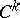category). Many problems concerning invariant tori are based on similar small denominator estimates.

In the discrete case, when one considers a mapping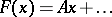, the analogous results also holds. In that case, the vector of eigenvaluesis in the Poincaré domain if the norm of all the eigenvaluesare all smaller or all greater than one. The complement is again called the Siegel domain. If the dimension, then this reduces to the unit circle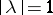.

In fact, in one-dimension the Siegel case is completely understood. So, assume thatand thatis not a root of unity. Writeand let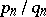be the continued fraction expansion of. If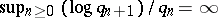, then the formal power series of the linearizing coordinates need not converge (Cremer's theorem). Around 1965, A.D. Bryuno proved that if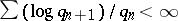, then this power series converges. In the late 1980s, Yoccoz proved the converse: if the Bryuno condition is not satisfied, then there exists a holomorphic diffeomorphism with linear part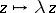which is not holomorphically linearizable. In 1994, Yoccoz was awarded the Field Medal for this achievement.

Ifis a rational mapping on the Riemann sphere, then Siegel domains appear in the Fatou–Sullivan classification theorem. Let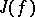be the closure of the set of repelling periodic points (cf. also Repelling set) and let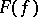be its complement.is also the set of points that have a neighbourhoodsuch thatforms a normal family. Many properties of these sets were already obtained by G. Julia and P. Fatou in the 1920s and 1930s. Using the measurable Riemann mapping theorem (cf. also Quasi-conformal mapping), D. Sullivan proved in the 1980s that each connected component ofis eventually mapped onto a periodic component, and that the periodic components can be of four types:

i) the basin of an attracting periodic point (where the eigenvalueof the linear part satisfies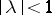);

ii) the basin of a parabolic periodic point (whenis a root of unity);

iii) a Siegel domain (whenhas norm one but is not a root of unity, but still the mapping is holomorphically linearizable on some neighbourhood of the fixed point);

iv) a Herman ring (when the component is an annulus on which the mapping is conjugate to a rotation).